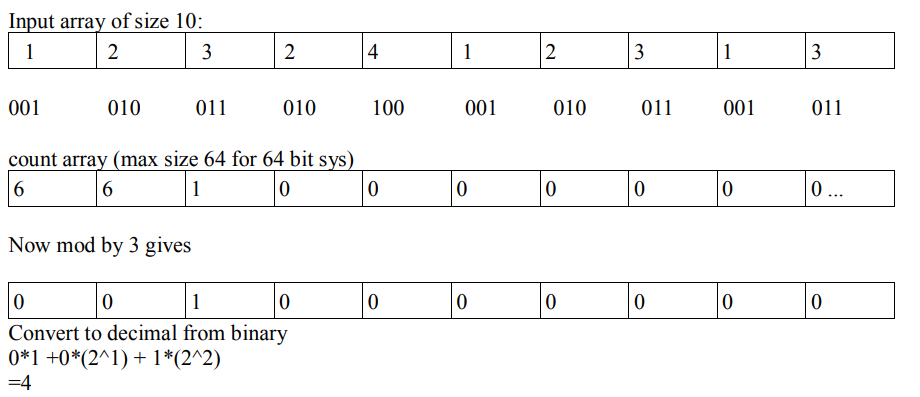# C++ program to find Unique Number using Bit Masking

Here, we are implementing a C++ program to find the unique number using bit masking.
Submitted by Saksham Bhayana, on December 11, 2018

Problem statement: C++ Program to find unique number in an array of n numbers in which except one (unique number) rest all are present thrice.

Constraints: n<10^5

Example:

```    Input:
10
1  2  3  2  4  1  2  3  1  3

Output:
4
```

Solution: Except 4 rest all have multiplicity of 3 in array. For instance 4 are represented as 100 in binary .Starting from left most bit is the first index of count array.Problem explanation:

1. Make a count array (initialize all elements to 0) to store the bits of each number in input array.
2. In each iteration access the input number and extract its bits to store in count array for instance if number is 6 which is 110 in binary, so 110 & 1 stores 0 at count, then right shift the number to obtain the next bit from left that is 11 & 1 stores 1 at count and similarly again >> number to get 1 again at count.
3. After each iteration count array is updated. Now since every element except one occurs thrice in input array, therefore bits at every index exist in the form of 3n and 3n +1.
4. So taking modulus by 3 leaves only unique number's bits in count array as remainders. This will give the binary number of the unique number.
5. Now convert binary to decimal by ∑ multiply (bit with 2^index at respective index).
6. Return the unique number in array.

Program:

```#include <iostream>
using namespace std;

int unique(int *arr,int n)
{
//array of size 64 for max 64 bit size
int count={0};

//count array stores bit of every number
for(int k=0;k<n;k++)
{
int i=0;
int num=arr[k];
while(num>0)
{
// extract bit
count[i]+=(num&1);
i++;
// right shift to get next leftmost bit
num=num>>1;
}
}

// starting from first index 2^0=1
int power=1;
int result=0;
for(int j=0;j<64;j++)
{
// take modulus of count array by 3
count[j] %=3;
result+=count[j]*power;
// binary to decimal operation
power=power<<1;
}
// if there is no unique number 0 is returned
return result;
}

int main()
{
int arr;
int n;
cout<<"Enter lenght of the array: ";
cin>>n;

cout<<"Enter array elements..."<<endl;
for(int c=0;c<n;c++)
{
cin>>arr[c];
}
cout<<unique(arr,n)<<" is the unique number in array.";

return 0;
}
```

Output

```Enter lenght of the array: 4
Enter array elements...
10 10 10 40
40 is the unique number in array.
```

TOP Interview Coding Problems/Challenges

Comments and Discussions

Ad: Are you a blogger? Join our Blogging forum.
Learn PCB Designing: PCB DESIGNING TUTORIAL

Languages: » C » C++ » C++ STL » Java » Data Structure » C#.Net » Android » Kotlin » SQL
Web Technologies: » PHP » Python » JavaScript » CSS » Ajax » Node.js » Web programming/HTML
Solved programs: » C » C++ » DS » Java » C#
Aptitude que. & ans.: » C » C++ » Java » DBMS
Interview que. & ans.: » C » Embedded C » Java » SEO » HR
CS Subjects: » CS Basics » O.S. » Networks » DBMS » Embedded Systems » Cloud Computing » Machine learning » CS Organizations » Linux » DOS
More: » Articles » Puzzles » News/Updates

© https://www.includehelp.com some rights reserved.